Next: 2-d problem with Dirichlet Up: Poisson's equation Previous: An example 1-d Poisson

## An example solution of Poisson's equation in 1-d

Let us now solve Poisson's equation in one dimension, with mixed boundary conditions, using the finite difference technique discussed above. We seek the solution of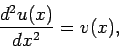(142)

in the region, with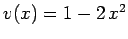. The boundary conditions at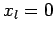and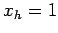take the mixed form specified in Eqs. (132) and (133). Of course, we can solve this problem analytically. In fact,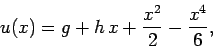(143)

where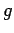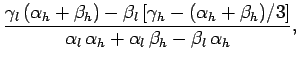(144)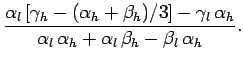(145)

Figure 62 shows a comparison between the analytic and finite difference solutions for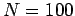. It can be seen that the finite difference solution mirrors the analytic solution almost exactly.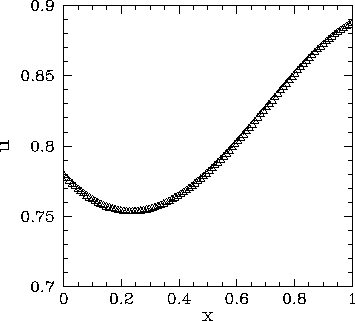Next: 2-d problem with Dirichlet Up: Poisson's equation Previous: An example 1-d Poisson
Richard Fitzpatrick 2006-03-29